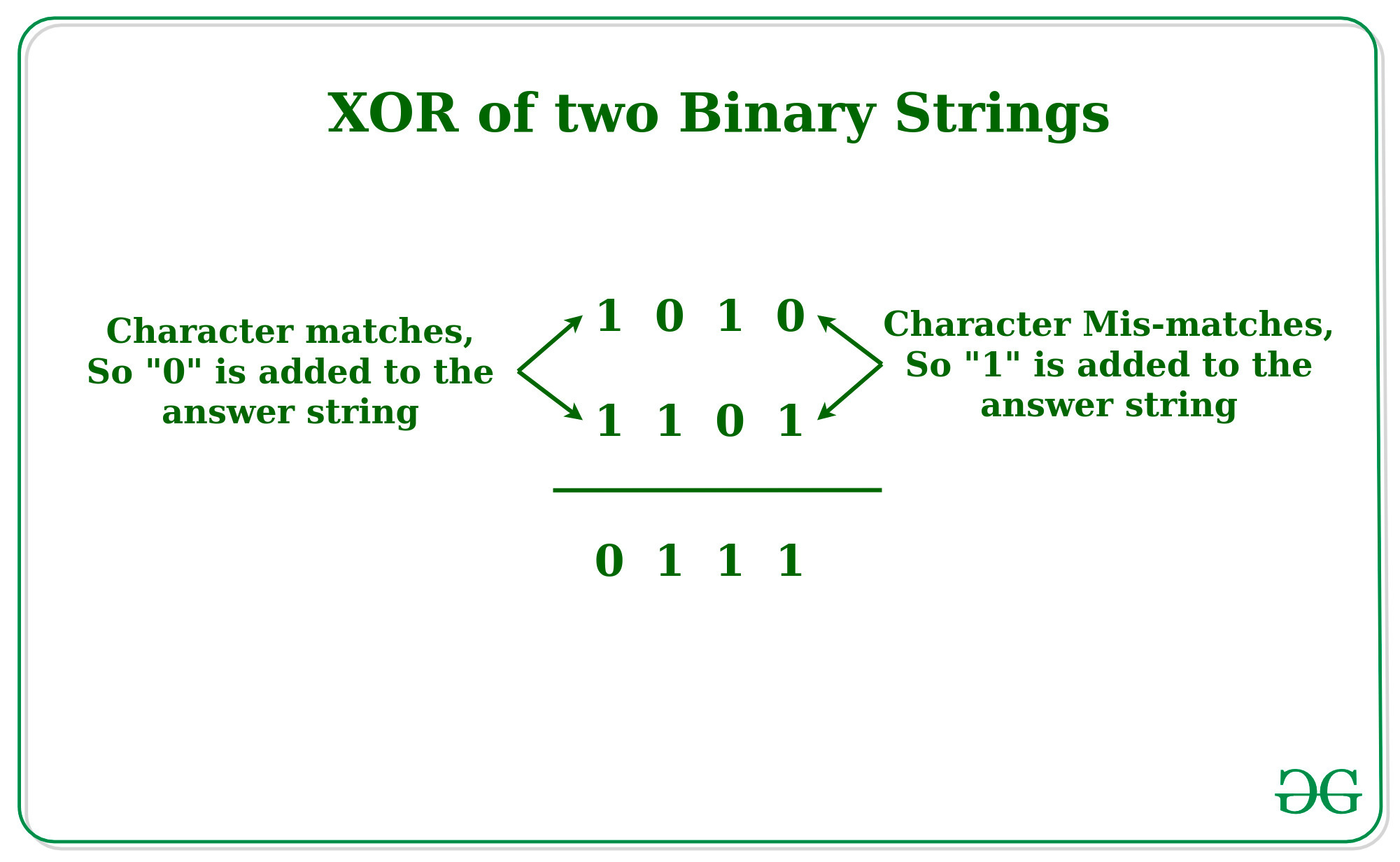# XOR of two Binary Strings

• Difficulty Level : Easy
• Last Updated : 30 Apr, 2021

Given two binary strings A and B of equal lengths, the task is to print a string which is the XOR of Binary Strings A and B.
Examples:

Input: A = “0001”, B = “0010”
Output: 0011
Input: A = “1010”, B = “0101”
Output: 1111

Approach: The idea is to iterate over both the string character by character and if the character mismatched then add “1” as the character in the answer string otherwise add “0” to the answer string to generate the XOR string.Below is the implementation of the above approach:

## C++

 `// C++ Implementation to find the``// XOR of the two Binary Strings``#include``using` `namespace` `std;` `// Function to find the``// XOR of the two Binary Strings``string xoring(string a, string b, ``int` `n){``string ans = ``""``;``    ` `    ``// Loop to iterate over the``    ``// Binary Strings``    ``for` `(``int` `i = 0; i < n; i++)``    ``{``        ``// If the Character matches``        ``if` `(a[i] == b[i])``            ``ans += ``"0"``;``        ``else``            ``ans += ``"1"``;``    ``}``    ``return` `ans;``}` `// Driver Code``int` `main()``{``    ``string a = ``"1010"``;``    ``string b = ``"1101"``;``    ``int` `n = a.length();``    ``string c = xoring(a, b, n);``    ``cout << c << endl;``}` `// This code is contributed by Surendra_Gangwar`

## Java

 `// Java Implementation to find the``// XOR of the two Binary Strings``import` `java.io.*;` `class` `GFG {``    ``// Function to find the``    ``// XOR of the two Binary Strings``    ``static` `String  xoring(String a, String b, ``int` `n){``    ``String ans = ``""``;``        ` `        ``// Loop to iterate over the``        ``// Binary Strings``        ``for` `(``int` `i = ``0``; i < n; i++)``        ``{``            ``// If the Character matches``            ``if` `(a.charAt(i) == b.charAt(i))``                ``ans += ``"0"``;``            ``else``                ``ans += ``"1"``;``        ``}``        ``return` `ans;``    ``}``    ` `    ``// Driver Code``    ``public` `static` `void` `main (String[] args)``    ``{``        ``String a = ``"1010"``;``        ``String b = ``"1101"``;``        ``int` `n = a.length();``        ``String c = xoring(a, b, n);``        ``System.out.println(c);``    ``}``}` `// This code is contributed by shubhamsingh10`

## Python3

 `# Python Implementation to find the``# XOR of the two Binary Strings` `# Function to find the``# XOR of the two Binary Strings``def` `xor(a, b, n):``    ``ans ``=` `""``    ` `    ``# Loop to iterate over the``    ``# Binary Strings``    ``for` `i ``in` `range``(n):``        ` `        ``# If the Character matches``        ``if` `(a[i] ``=``=` `b[i]):``            ``ans ``+``=` `"0"``        ``else``:``            ``ans ``+``=` `"1"``    ``return` `ans` `# Driver Code``if` `__name__ ``=``=` `"__main__"``:``    ``a ``=` `"1010"``    ``b ``=` `"1101"``    ``n ``=` `len``(a)``    ``c ``=` `xor(a, b, n)``    ``print``(c)`

## C#

 `// C# Implementation to find the``// XOR of the two Binary Strings``using` `System;` `class` `GFG{``    ``// Function to find the``    ``// XOR of the two Binary Strings``    ``static` `string` `xoring(``string` `a, ``string` `b, ``int` `n){``    ``string` `ans = ``""``;``        ` `        ``// Loop to iterate over the``        ``// Binary Strings``        ``for` `(``int` `i = 0; i < n; i++)``        ``{``            ``// If the Character matches``            ``if` `(a[i] == b[i])``                ``ans += ``"0"``;``            ``else``                ``ans += ``"1"``;``        ``}``        ``return` `ans;``    ``}``    ` `    ``// Driver Code``    ``static` `public` `void` `Main ()``    ``{``        ``string` `a = ``"1010"``;``        ``string` `b = ``"1101"``;``        ``int` `n = a.Length;``        ``string` `c = xoring(a, b, n);``        ``Console.WriteLine(c);``    ``}``}` `// This code is contributed by shubhamsingh10`

## Javascript

 ``

Output:

`0111`

My Personal Notes arrow_drop_up